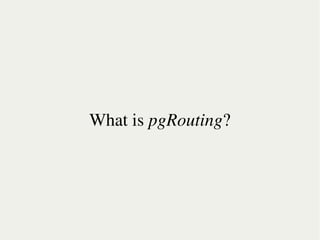Successfully reported this slideshow.×
1 of 48

Shortest path search for real road networks and dynamic costs with pgRouting

8

Share

This presentation will show the inside and current state of pgRouting
development. It will explain the shortest path search in real road
networks and how the data structure is important for getting better
routing results. We will show how you can improve the quality of the search with dynamic costs and make the result look closer to the reality. We will demonstrate the way of using pgRouting together with other Open Source tools. Also you will learn about difficulties and limitations of implementing routing functionality in GIS applications, the difference between algorithms and their performance.

pgRouting is an extension of PostgreSQL and PostGIS. A predecessor of
pgRouting - pgDijkstra, written by Sylvain Pasche from Camptocamp, was
extended by Orkney (Japan) and renamed to pgRouting, which now is a part of the PostLBS project.

pgRouting can perform:
* shortest path search (with 3 different algorithms)
* Traveling Salesperson Problem solution (TSP)
* driving distance geometry calculation

See all

See all

Shortest path search for real road networks and dynamic costs with pgRouting

1. 1. Shortest path search for real road networks     Anton Patrushev
2. 2. What is pgRouting?
3. 3.     Shortest path
4. 4.     TSP and DD
5. 5.     VRP (DARP)
6. 6. Real road networks How do they look like?
7. 7. Like this...
8. 8. ...or like this...
9. 9. ...or sometimes like this.
10. 10. What makes them real? traffic lights signs road marking
11. 11. How pgRouting can help here?
12. 12. gid, source, source target, cost, reverse_cost, x1, y1, x2, y2, rule, to_cost target
13. 13. Traffic lights make you slower. It means we should increase costs.
14. 14. B C 1min A D cost(A)=cost(A)+30sec cost(B)=cost(B)+30sec cost(C)=cost(C)+30sec     cost(D)=cost(D)+30sec
16. 16.
17. 17. cost(A)=length(A) reverse_cost(A)=∞ A
18. 18. Sometimes the costs have different meaning.
19. 19. cost(A)=length(A)/2 reverse_cost(A)=length(A)*2 cost(B)=length(B)*3 reverse_cost(B)=length(B)/3 B   A
20. 20. Turn restrictions obviously restrict turns.
21. 21. B C 1min A D cost(A)=cost(A)+1min cost(B)=cost(B)+1min rule(A)="D" cost(C)=cost(C)+1min to_cost(A, D)=∞     cost(D)=cost(D)+1min
22. 22. Road type signs can be used for cost calculation.
23. 23. Not only types, but also conditions.
25. 25. It separates lanes, ...
26. 26. ...specifies road types, ...
27. 27. ... and restrictions. C A D B E rule(D)="A, B"   to_cost(D)=∞
28. 28. And you know what's cool about pgRouting?
29. 29. All costs are dynamic. Which is opposite to pre-calculated.
30. 30. If the road is closed, ...
31. 31. ... there is an accident, ...
32. 32. ... a sign with restrictions limited in time, ...
33. 33.   ... bad weather conditions, ...
34. 34. ... or an obstacle, ...